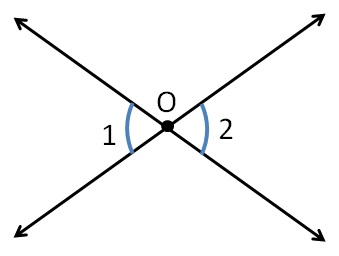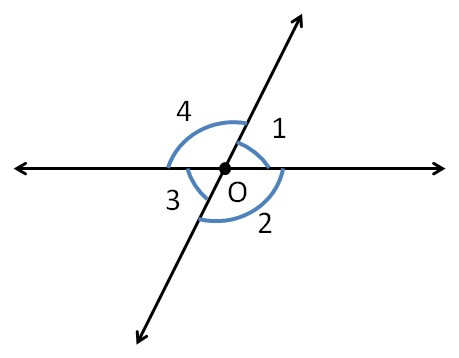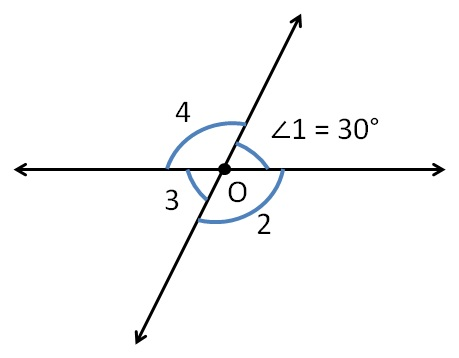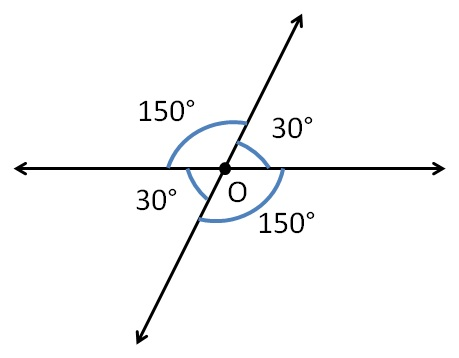Adjacent Angles, Linear Pair of angles, Vertically Opposite angles

Chapter 5 Class 7 Lines and Angles
Concept wise

When two lines intersect,

angles opposite to each other vertically opposite angles.

Vertically opposite angles have

• Common vertex
• And are opposite to each other

Here,∠1 & ∠2 have common vertex O

& are opposite to each others

So, they are vertically opposite angles.

Also,

Vertically opposite angles are equal

∴ ∠1 = ∠2

Let’s take this figureHere,

∠1 & ∠3 are vertically opposite angles

∴ ∠1 = ∠3

∠2 & ∠4 are vertically opposite angles

∴ ∠2 = ∠4

Let’s do some questions

Find ∠ 2, ∠3, ∠4Now,

∠3 & ∠1 are vertically opposite angles

∴ ∠3 = ∠1

∠3 = 30°

Also,  ∠2 & ∠4 are vertically opposite angles

∴ ∠2 = ∠4      ......(1)Now,

∠1 & ∠2 are linear pair

∴ ∠1 + ∠2 = 180°

30° + ∠2 = 180°

∠2 = 180° − 30°

∠2 = 150°From (1)

∠4 = ∠2

∠4 = 150°

So, our figure looks like

Learn in your speed, with individual attention - Teachoo Maths 1-on-1 Class